# Functions + expression of a variable from the formula - math problems

#### Number of problems found: 292

• The notebook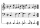After rising by 40%, the notebook cost 10.50 euros. How much did this notebook cost if it increased in price by only 20% instead of 40%?
• Annual growthThe population has grown from 25,000 to 33,600 in 10 years. Calculate what was the average annual population growth in%?
• Harmonic mean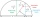If x, y, z form a harmonic progression, then y is the harmonic mean of x and z. Find the harmonic mean of the numbers 6 and 5.
• Cuboid edges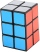The lengths of the cuboid edges are in the ratio 2: 3: 4. Find their length if you know that the surface of the cuboid is 468 m2.
• Three groupsIn the company, employees are divided into three groups. In the first group, which includes 12% of the company's total number of employees, the average salary is CZK 40,000, in the second group CZK 35,000, in the third group CZK 25,000. The average salary
• Sphere cutA sphere segment is cut off from a sphere k with radius r = 1. The volume of the sphere inscribed in this segment is equal to 1/6 of the segment's volume. What is the distance of the cutting plane from the center of the sphere?
• Railway embankmentThe railway embankment section is an isosceles trapezoid, the sizes of the bases of which are in the ratio 5: 3. The arms have a length of 5 m, and the height of the embankment is 4.8 m. Calculates the size of the embankment section area.
• DecibelBy what percentage does the sound intensity increase if the sound intensity level increases by 1 dB?
• Vertical rodThe vertical one-meter-long rod casts a shadow 150 cm long. Calculate the height of a column whose shadow is 36 m long at the same time.
• The string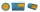They cut 113 cm from the string and divided the rest in a ratio of 5: 6.5: 8: 9.5. The longest part measured 38 cm. Find the original length of the string.
• Sphere in coneA sphere is inscribed in the cone (the intersection of their boundaries consists of a circle and one point). The ratio of the surface of the ball and the contents of the base is 4: 3. A plane passing through the axis of a cone cuts the cone in an isoscele
• Integer sidesA right triangle with an integer length of two sides has one leg √11 long. How much is its longest side?
• Equilateral coneWe pour so much water into a container that has the shape of an equilateral cone, the base of which has a radius r = 6 cm, that one-third of the volume of the cone is filled. How high will the water reach if we turn the cone upside down?
• Powerplant chimneyFrom the building window at the height of 7.5 m, we can see the top of the factory chimney at an altitude angle of 76° 30 ′. We can see the chimney base from the same place at a depth angle of 5° 50 ′. How tall is the chimney?
• CoinsThe money - coins are minted from the hardest bronze, which contains copper and tin in a ratio of 41: 9. How much copper and tin are in 2kg of bronze money?
• Chord of triangle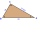If the whole chord of the triangle is 14.4 cm long, how do you calculate the shorter and longer part?
• Coils of transformerThe primary coil of the transformer has 400 turns, a current of 1.5 A passes through it and is connected to a voltage of 220 V. For the secondary coil, find the voltage, current, and a number of turns if the transformation ratio k = 0.1.
• DodecagonCalculate the size of the smaller of the angles determined by lines A1 A4 and A2 A10 in the regular dodecagon A1A2A3. .. A12. Express the result in degrees.
• Twice of radiusHow many times does the surface of a sphere decrease if we reduce its radius twice?
• Lookout towerHow high is the lookout tower? If each step was 3 cm lower, 60 more of them were on the lookout tower. If it were 3 cm higher again, it would be 40 less than it is now.

Do you have an interesting mathematical word problem that you can't solve it? Submit a math problem, and we can try to solve it.

We will send a solution to your e-mail address. Solved examples are also published here. Please enter the e-mail correctly and check whether you don't have a full mailbox.

Please do not submit problems from current active competitions such as Mathematical Olympiad, correspondence seminars etc...

Functions - math problems. Expression of a variable from the formula - math problems.